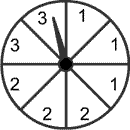Skill 26G
Probability & Outcomes
Even though there are eight sections on the spinner, there are only 3 possible outcomes since the numbers 1, 2 and 3 are the only options. Since there are three 1's and three 2's and only two 3's, then it is equally likely that the spinner could land on 1 or 2 and less likely that it could land on 3.
Answer the following questions about the spinner?1. What are the possible outcomes of this spinner? 2. Which outcomes are equally likely?